.NET C# 教程初级篇 1-1 基本数据类型及其存储方式

全文目录

（博客园）.NET Core Guide

（Github）.NET Core Guide

C# 中两种数据存储方式

C# 中，数据在内存中的存储方式主要分为在堆中存储和栈中存储。我们之前提到的值类型就是存储在栈中，引用类型的数据是存储在堆中，而数据是在栈中。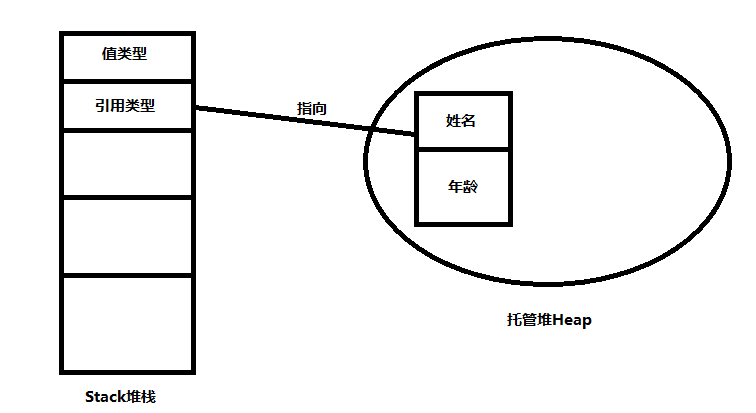托管堆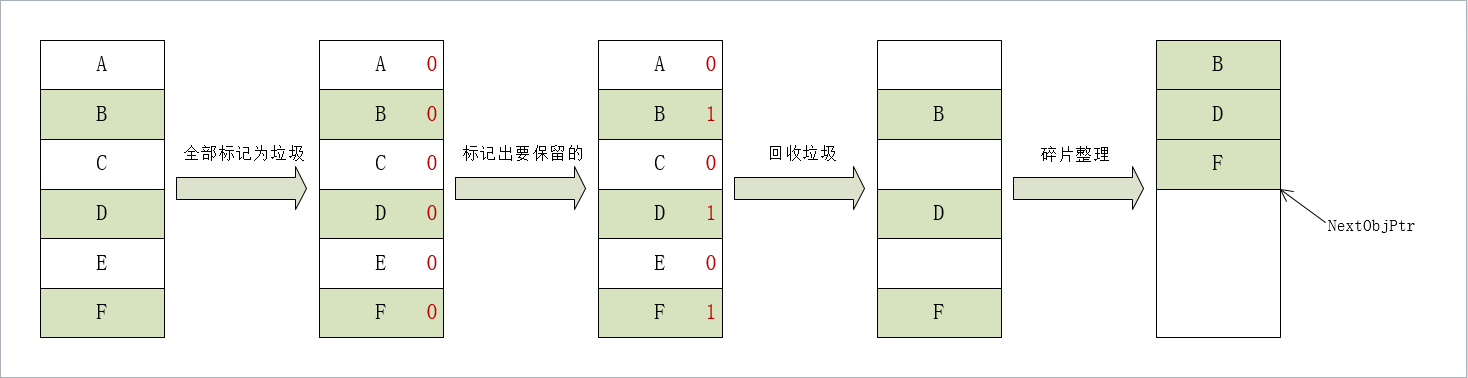常见的几种数据类型

• 字符类型：char字符类型，代表无符号的16位整数，对应的可能值是ASCⅡ码,你可以上网搜索ASCⅡ码的内容
• 整数类型：常用的一般有：byte，short，int，long。各代表8位、16位、32位、64位整型。占用内存分别为（位数/8）字节。范围则是 +-(位数)个1组成的二进制的十进制数/2。例如byte的范围则是11111111转十进制后除以2取反，即-127~128。范围绝对值之和为256。
• 浮点类型：float, double, decimal：浮点类型，分别代表32位、64位、128位浮点类型。通常默认类型是double，如果需要指定float类型，需要1.3f，decimal类型则指定1.3m。浮点型存在的问题是精度的损失，并不一定安全。
• 布尔类型：bool类型是一个二进制中的0和1，各代表了false和true。只存在两个值。
• 字符串类型：string本质是一种语法糖，作为字符类型的数组引用(指针)存在，也是String类的简写
• 委托类型：delegate用于绑定函数，为引用类型的一种，将函数参数化为变量。本质上就是C++中的函数指针。
• 数组：继承自Array类，属于任意类型的一种集合，但不同于集合，大小必须被初始化。在内存中是一段连续的内存空间，但是不是值类型。

数据的存储方式

进制

$\sum_{i=0}^{-m}K_i*2^i$

$\sum_{i=0}^{-m}K_i*r^i$

int a = 0b101011;//二进制
int b = 035167;//八进制
int a = 0xD2F3;//十六进制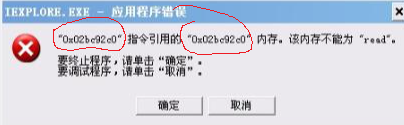1011011011转八进制的过程，先添0补足长度为3的倍数，001011011011，分组001|011|011|011，则表示为1333，十六进制和N进制转2进制希望读者自己尝试解决。

// value为需转换的R进制数，以字符串表示，fromBase为需转换的进制
Convert.ToInt32(string value, int fromBase)：

// value为需转换的十进制数，toBase为需转换的进制
Convert.ToString(int value, int toBase);

正负数存储形式及四种码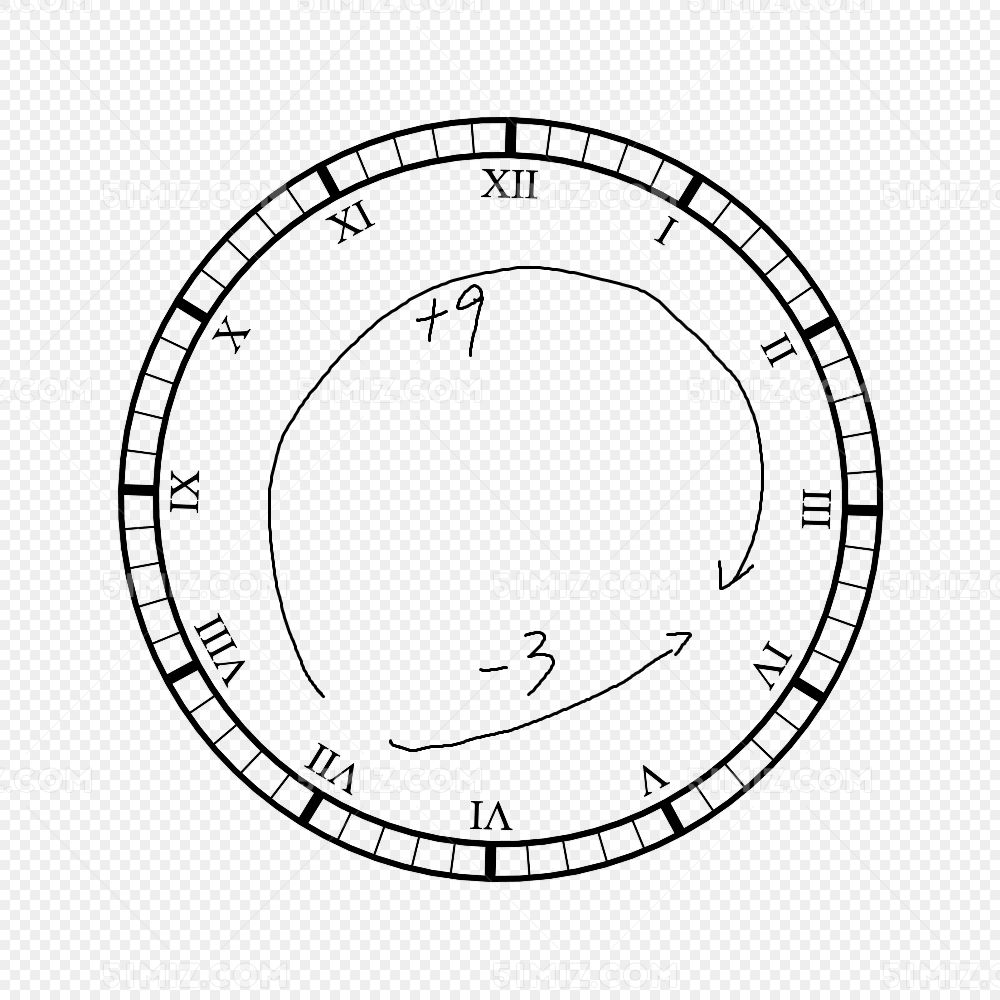$\forall x>0,\forall y>0;\\ y_补=max-y_原+1\\ x+y_补 = x-y_原+max+1\\ 因此很显然x-y = x+y_补=(x-y_原+max+1)\%(max+1)得证 \\$

$[A+B]_补 = （A_补+B_补）mod M \\ \\ [A-B]_补 = （A_补+[-B]_补）mod M$

*数据的存储方式（选看）

• 小端存储就是低位字节排放在内存的低地址端，高位字节排放在内存的高地址端。
• 大端存储就是高位字节排放在内存的低地址端，低位字节排放在内存的高地址端。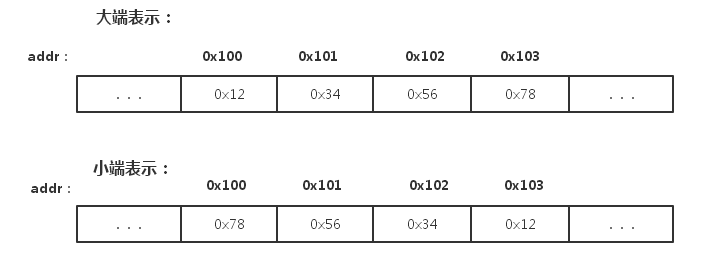C#中定义变量的方式及数据转换的方法

int number = 5;//定义一个32位整数类型
bool b = true;//定义
//注意看以下两条，string定义的字符串必须为双引号，而char使用单引号并且只允许输入一个字符
string str = "test";
char a = 'a';
//记得后缀
float f = 1.3f;
decimal d = 1.5m;

string a = "15";
int b = int.Parse(a);//显式转换
b = (int)a;//强制转换
b = Convert.ToInt32(a);//显式转换，较常用
double d = 1.5;
b = d;//隐式转换

int a = -3;//补码为100
uint b = a;//b=8

数组

//伪代码，T为类型，n为大小
T [] t = new T[n]；
//定义一个整型数组
int [] a = new int ;
//你也可以选择初始化的方式定义
int [] b = new int [] {1,2,3,4,5};
//或
int [] c = new int {1,2,3,4,5};
//数组的访问，从0开始索引
Console.WriteLine(b);

//伪代码，T为类型，m,n为大小
T [,] t = new T[m,n]；

运算符及规则重载

基础的运算符

• +-*/：对应数学中的加减乘除。
• %： 求余运算，a%b指a除以b的余数。
• & | ~ ^ ：分别为按位与、按位或、按位取反、按位异或
• <<、>>：左右移位运算符,例如0010 –> 0100
• ？：三元判断运算符

^是异或,result=1110,就是说异是不同返回1，相同是0，或就是只要有1就返回1。

&是与, result=0001,也就是相同返回1，不同为0

|是或, result=1111,除了两个都为0，否则返回1

~称为按位取反，我们表示符号是用四个0表示，运算规则就是正数的反码，补码都是其本身的源码，

>>称为右移,右移一位流程如下 0000 1001 –> 0000 0100 = 4

<< 称为左移,左移一位流程如下 0000 1001 –> 0000 10010 = 18

运算符的重载

// T是修改类型的返回值
public static T operator +(D d1,D d2)
{
return something;
}

*结构体(选看)

struct S
{
int a;
long b;
int c;
}
struct SS
{
int a;
int b;
long c;
}

• S：a(4 byte + 4 free) –> b(8 byte) –> c(4 byte + 4 free)，共计24字节
• SS：a(4 byte)b(4 byte) –> c(8 byte)，共计16字节

struct S
{
char a;
long b;
string c;
}
//函数中创建
S s = new S();
s.a = 'a';
s.b = 15;
s.c = "I Love .NET Core And Microsoft"

a(8) –> c(8) –> b(8)。

练习题

理论分析题

• 计算出int和long的数值范围
• 为什么在大部分提供科学计算或编程语言会存在精度问题？例如浮点数2.5在任何一种采用二进制计算的编程语言中也不是一个精确值？或者说如果我们展开浮点数的所有精确位，最后的几位小数并不是0？（较难）
• 为什么引用类型即使不存储内容也需要内存空间？
• 试说明引用类型和值类型的优缺点
• 数组为什么需要初始化大小？如果是多维数组，不指定列宽可以吗？

计算题

• 求123.6875的二进制、八进制、十六进制表达式。
• $$(11011.101)_2$$二进制小数转换为十进制。
• a=5，b=8，试手算a&b，a|b，a^b，a<<1， b>>1
• 若a=12，试手算~a
• 若a为8位二进制，试着写出将a的高四位取反，第四位不变的运算表达式
• int a = 15，试求a+int.MaxValue的值

编程题

• 请学习指针内容以及C#unsafe调试，试着不使用索引进行数组的读取。
• 将字符串”15”转成整数？
• 使用运算符重载，计算向量的加减和点乘（内积）

Reference

《C# in Depth》—— Jon Skeet

《计算机组成原理》——唐朔飞

C#托管堆和垃圾回收（GC）

C# Heap(ing) Vs Stack(ing) In .NET

C# 大端与小端（因为大小端引起的奇怪问题）

联系方式